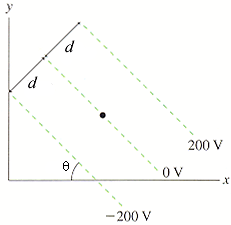# Problem: What are the magnitude and direction of the electric field at the dot in Figure below, in which d = 5 cm and theta = 47°?in units of V/m (degree measured counterclockwise from the +x-axis)

###### FREE Expert Solution

Electric field:

$\overline{){\mathbf{E}}{\mathbf{=}}{\mathbf{-}}\frac{\mathbf{d}\mathbf{V}}{\mathbf{d}\mathbf{r}}}$

Substituting:

E = (-200 - 200)/9(5 + 5) × 10-2] = - 400 V/m###### Problem Details

What are the magnitude and direction of the electric field at the dot in Figure below, in which d = 5 cm and theta = 47°?in units of V/m (degree measured counterclockwise from the +x-axis)